# MP Board Class 10th Maths Solutions Chapter 3 Pair of Linear Equations in Two Variables Ex 3.6

In this article, we will share MP Board Class 10th Maths Book Solutions Pair of Linear Equations in Two Variables Ex 3.6 Pdf, These solutions are solved subject experts from the latest edition books.

## MP Board Class 10th Maths Solutions Chapter 3 Pair of Linear Equations in Two Variables Ex 3.6

Question 1.
Solve the following pairs of equations by reducing them to a pair of linear equations: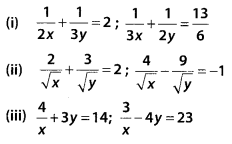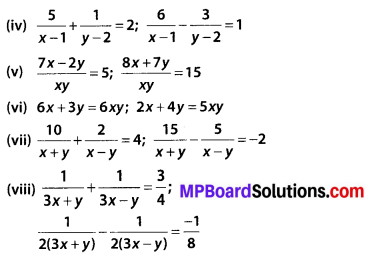Solution:
(i) we have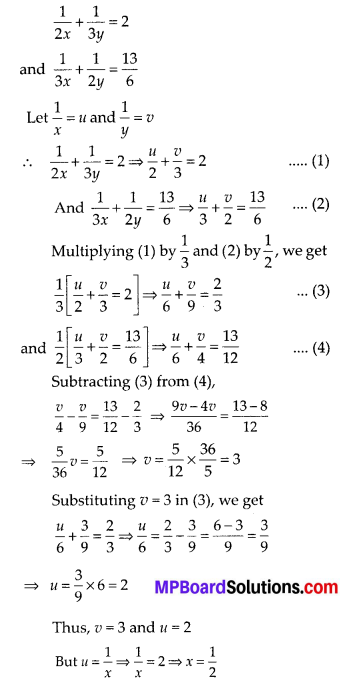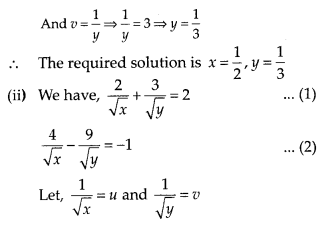Equations (1) and (2) becomes,
2u + 3v = 2 ⇒ 2u + 3v – 2 = 0 … (3)
4u – 9v = -1 ⇒ 4u – 9v + 1 = 0 … (4)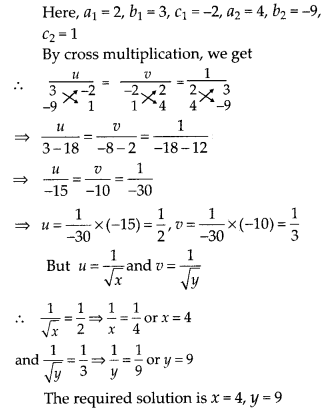(iii)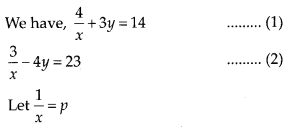The equations (1) and (2) becomes,
4p + 3y = 14 ⇒ 4y + 3y -14 = 0 …. (3)
3p – 4y = 23 ⇒ 3p – 4y – 23 = 0 …. (4)
Here, a1 = 4, b1= 3, c1 = —14, a2 = 3, b2 = – 4, c2 = -23
Solving (3) and (4) by cross multiplication method, we get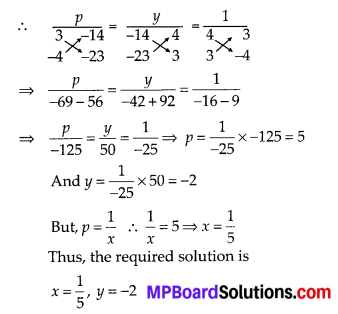(iv)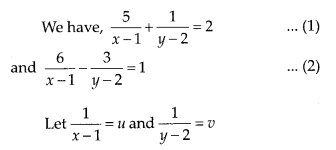Equations (1) and (2) can be expressed
5u + v = 2 ⇒ 5u + v – 2 = 0 …. (3)
6u – 3v = 1 ⇒ 6u – 3v – 1 = 0 …. (4)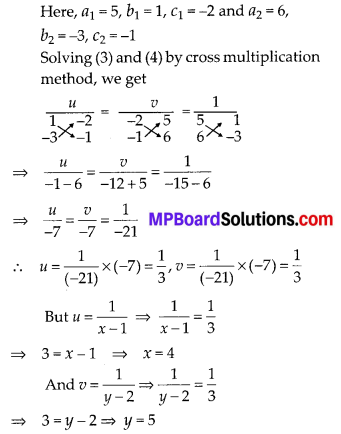Thus, the required solution is x = 4, y = 5

(v)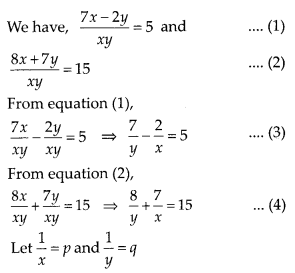∴ Equation (3)and (4)can be expressed as:
7q – 2p – 5 = 0 … (5)
8q + 7p -15 = 0 … (6)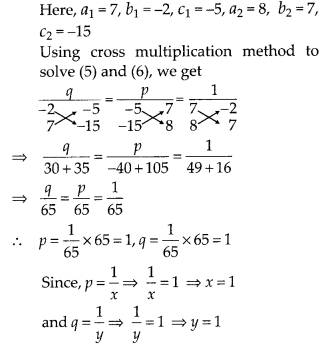Thus, the required solution is x = 1, y = 1(vi) We have, 6x + 3y = 6xy …. (1)
2x + 4y = 5xy … (2)
From (1) , we get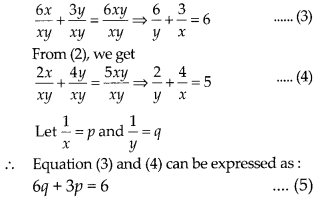2q + 4p = 5 ….(6)
Multiply (6) by 3, we get
6q +12p = 15 … (7)
Subtracting (5) from (7) , we get
6q + 12p – 6q – 3p = 15 – 6
9p = 9 ⇒ p = 1
Now, Substituting, p = 1 in (5) , we get
6q + 3(1) = 6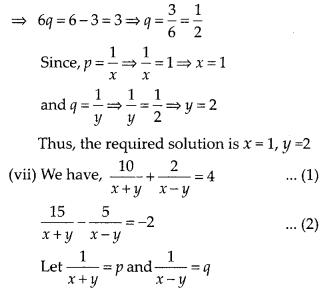∴ Equations (1) and (2) can be expressed as :
10p + 2q = 4 = ⇒ 10p + 2q – 4 = 0 …(3)
15p – 5q = -2 ⇒ 15p – 5q + 2 = 0 … (4)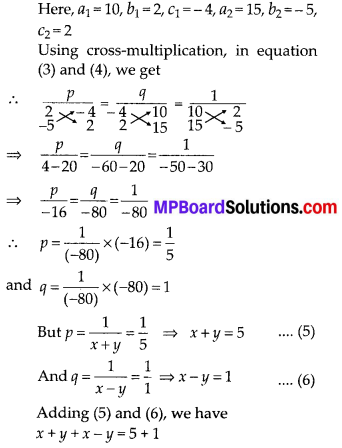⇒ 2x = 6 ⇒ x = 3
Now, substituting the value of x in equation (5) , we get
3 + y = 5 ⇒ y = 2
Thus, the required solution is x = 3, y = 2

(viii)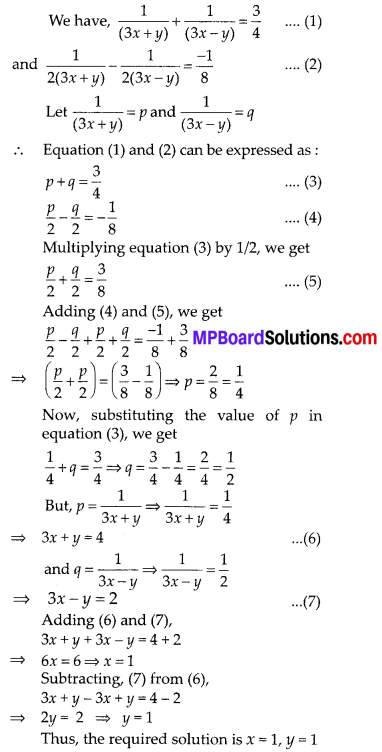Question 2.
Formulate the following problems as a pair of equations, and hence find their solutions:
(i) Ritu can row downstream 20 km in 2 hours, and upstream 4 km in 2 hours. Find her speed of rowing in still water and the speed of the current.
(ii) 2 women and 5 men can together finish an embroidery work in 4 days, while 3 women and 6 men can finish it in 3 days. Find the time taken by 1 woman alone to finish the work, and also that taken by 1 man alone.
(iii) Roohi travels 300 km to her home partly by train and partly by bus. She takes 4 hours if she travels 60 km by train and the remaining by bus. If she travels 100 km by train and the remaining by bus, she takes 10 minutes longer. Find the speed of the train and the bus separately.
Solution:
(i) Let the speed of Ritu in still water = x km/hr
and the speed of the current = y km/hr
∴ Downstream speed = (x + y) km/hr
Upstream speed = (x – y)km/hr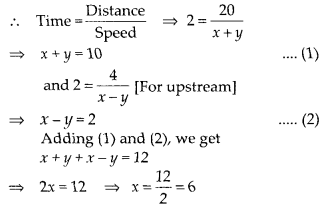Substituting the value of x in equation (1), we get
6 + y = 10 ⇒ y = 10 – 6 = 4
Thus, speed of rowing in still water = 6 km/hr, speed of current = 4 km/hr

(ii) Let the time taken to finish the task by one woman alone = x days
and the time taken to finish the task by one man alone = y days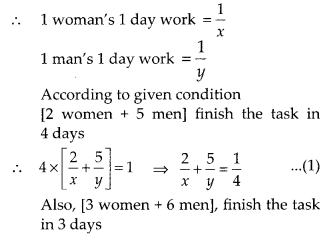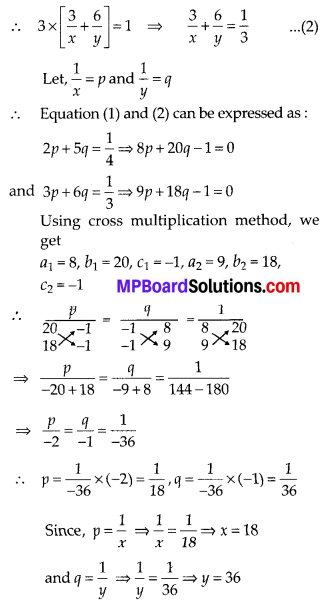∴ 1 man can finish the work in 36 days and 1 woman can finish the work in 18 days,

(iii) Let the speed of the train = x km/hr
and the speed of the bus = y km/hr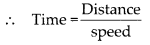Case I: Total journey = 300 km
Distance travelled by train = 60 km
Distance travelled by bus = (300 – 60)km
= 240 km
∵ Total time taken = 4 hours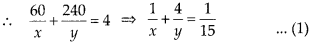Case II: Distance travelled by train = 100 km
Distance travelled by bus = (300 – 100)km
= 200 km
Total time = 4 hrs 10 mins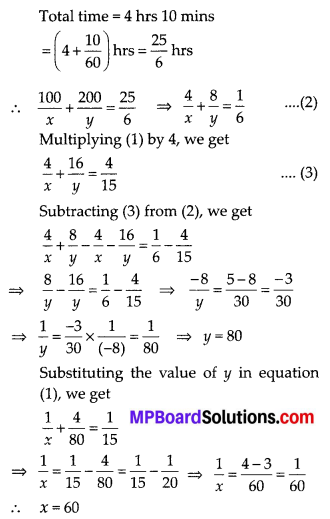Thus, speed of the train = 60 km/hr
and speed of the bus = 80 km/hr